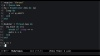In Progress
Unit 1, Lesson 1
In Progress

# Enumerable QueueHave you ever been working with a collection object from some third-party API, and been surprised to discover that it doesn’t support Ruby’s ubiquitous `#each` protocol for iterating over elements? Let alone the full `Enumerable` method set?

In this episode, you’ll learn how to use Ruby’s `Enumerator` library to quickly put a fully-functional `Enumerable` wrapper over any type of object.

Video transcript & code

We've been talking about the Queue class lately. Surprisingly for a Ruby collection class, queues do not implement the #each method, nor do they include Enumerable. Let's say we'd like a queue that does support `Enumerable`.

This seems like a great opportunity to look at another use of the versatile Enumerator class. We've played with Enumerator a few times now, in Episode #59, Episode #60, and Episode #78. Usually, we've used the #to_enum method to convert an object into an `Enumerator`.

``````require 'pathname'
p = Pathname.new("/usr/local/bin")
ascender = p.to_enum(:ascend)
``````

This time around we're going to construct an Enumerator "from scratch", so to speak. To do so, we make a new `Enumerator` and give the constructor a block. This block will yield in something called a "yielder" object, which we'll abbreviate to `y`. In order to produce values for the `Enumerator` to iterate over, we need to feed values into this yielder.

Inside the block we start an infinite loop, and in each iteration we shift a value off of the queue and into the yielder. And that's enough to get us started. We now have an enumerable queue, which is a convenient way to build consumer threads. Here's one that consumes numbers from the queue and doubles them. When it receives the special `:stop` flag instead of a number, it breaks out of the loop.

We can demonstrate this by queuing up some numbers followed by a :stop signal. When we allow the `doubler` thread to proceed by calling #join on it, we can see that it outputs the doubled values before finishing.

``````require 'thread'
q = Queue.new
eq = Enumerator.new do |y|
loop do
y << q.shift
end
end

eq.each do |n|
break if :stop == n
puts n + n
end
end

q << 1
q << 2
q << 3
q << :stop
doubler.join
# >> 2
# >> 4
# >> 6
``````

This lets us use all that Enumerable and Enumerator goodness on a threadsafe queue. For instance, we can record message counts automatically:

``````require 'thread'
q = Queue.new
eq = Enumerator.new do |y|
loop do
y << q.shift
end
end

eq.with_index do |n, i|
break if :stop == n
puts "#{n + n}\t(msg# #{i})"
end
end

q << 2
q << 4
q << 6
q << :stop
doubler.join
# >> 4  (msg# 0)
# >> 8  (msg# 1)
# >> 12 (msg# 2)
``````

Or we could easily skip through the queue discarding items until we get to a message we're looking for.

``````require 'thread'
q = Queue.new
eq = Enumerator.new do |y|
loop do
y << q.shift
end
end

_, payload = eq.detect{|m| m.first == :priority}
end

q << [:ping]
q << [:log]
skipper.join
# >> Priority message: Red alert!
``````

One way in which this `Enumerator` is a little bit hobbled compared to other `Enumerable` objects is that it has no concept of its own size. In Ruby 2.0 and above we can remedy this by passing in a proc object to the Enumerator constructor. It will call this proc to determine its size without actually iterating through the whole queue.

Let's use the queue's size method to report the size of the enumerable queue.

``````RUBY_VERSION                    # => "2.0.0"
q = Queue.new
eq = Enumerator.new(->{q.size}) do |y|
loop do
y << q.shift
end
end

_, payload = eq.detect{|m| m.first == :priority}
end

q << [:ping]
q << [:log]